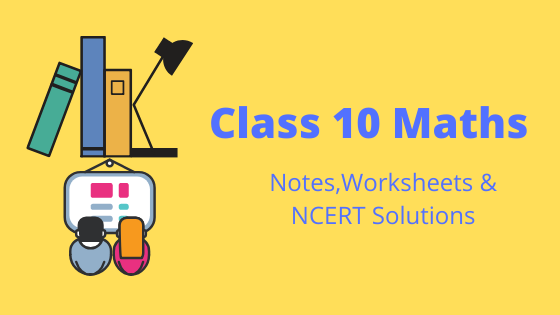# Notes and NCERT Solutions for Class 10 Maths

•• Welcome to our class 10 Maths page. In this page you can find class 10 Maths Ncert solutions , notes, assignments, worksheets,Practice questions etc. You can share the link to free Class 10 NCERT Maths solutions with your classmates as all study material is free of cost.

• First read and understand the notes.
• Try to go through the solved examples of the chapter you are reading
• Now it is the time to solve unsolved assignments and worksheets. You will find plenty of them here or on the respective chapter page.
You can check out syllabus at CBSE syllabus for class 10
All the best for better learning of your maths concepts. Hope we helped you when you were looking for class 10 Maths notes .

## Chapter 1 : Real Numbers

This chapter is about Euclid division algorithm and the Fundamental Theorem of Arithmetic for Positive Integers, Rational and Irrational Numbers NCERT Solutions

## Chapter 2 : Polynomials

This chapter is about Types of Polynomials, Zeroes of the Polynomials,Division algorithm for Polynomials,Relation between coefficient and zeros of the Polynomial NCERT Solutions

## Chapter 3: Pair of Linear Equations in Two Variables

This chapter is about Linear equations, Graphical Representation of Linear equation in one and two variables, Algebraic Solution of system of Linear equation in two variables, Simultaneous pair of Linear equation in Three Variable, Steps to solve the equations NCERT Solutions

## Chapter 5: Arithmetic Progression

Concept of Arithmetic Progression is introduced and we discussed about nth term of Arithmetic Progression and Sum of n item in Arithmetic Progression NCERT Solutions

## Chapter 6: Similar Triangles

In this chapter,we discussed about Similar Figures, Basic Proportionally Theorem, Criteria for Similarity of Triangles, Different Criterion for similarity of the triangles.We also found the Areas of Similar Triangles NCERT Solutions

## Chapter 7: Coordinate Geometry

We discussed about Distance and Sector Formula in Coordinate Geometry.We also check out how to calculate the area of triangle using coordinate of the vertices.We also application of these formula to solve general problem of area in Coordinate geometry NCERT Solutions

## Chapter 8: Introduction to trigonometry

Concept of Trigonometry and Trigonometric ratios are introduced. We also discussed the Trigonometric Ratios of Common angles and Trigonometric ratios of complimentary angles.Trigonometric identities are also touched upon NCERT Solutions

## Chapter 9: Trigonometry Application

In this chapter, We discussed the application of Trigonometric ratios in various areas. NCERT Solutions

## Chapter 10: Circles

This chapter we introduced meaning of a tangent to a circle. We discussed two important theorems related to circle which are the tangent to a circle is perpendicular to the radius through the point of contact and the lengths of the two tangents from an external point to a circle are equal
NCERT Solutions

## Chapter 11: Areas Related to Circles

< This chapter we introduced the formula for the circumference and area of the circle. We also discussed about arc, sector and segment in the circle and how to calculate the length and areas of these. NCERT Solutions

## Chapter 12: Surface Area and Volume

In this chapter we discussed about determining the surface area and volume of an object formed by combining any two of the basic solids, namely, cuboid, cone, cylinder, sphere and hemisphere. Formula for Frustum of a Right Circular Cone is also introduced NCERT Solutions

## Chapter 13: Statistics

This chapter introduces the concept of Un-group and group Frequency table .How to calculate the mean, mode and median for grouped frequency table.Empirical Formula between Mode, Mean and Median is also given NCERT Solutions

## Chapter 14: Probability

This chapter is about The difference between experimental probability and theoretical probability. Theoretical probability formula is also introduced. We also saw the probabilities of some important events NCERT Solutions

## Frequently asked Questions on CBSE Class 10 Maths

### How many chapters are there in Class 10 Maths?

There are total 14 chapters in Class 10 Science as per the rationalized Syllabus. All the chapters will come in Board examination

### What is the best books for Class 10 Maths?

Couple of book
(a)NCERT Class 10 Maths textbook
(b)NCERT Class 10 Maths Exemplar
(c)RS Agarwal and RD Sharma
(d)Previous Year Questions papers

### Which is the easiest chapter of Class 10 Maths NCERT Text book?

Real numbers and Polynomials are the easiast chapter

### Is Class 10 Maths NCERT Text book Sufficient for CBSE Exams?

It is sufficient if you go through all the questions in the NCERT text book thoroughly and practice them all.However to enhance your Maths skill , you can go for NCERT exemplar and other books

### what is the best way to learn the concept in Class 10 Maths?

First go through all the Notes and solved examples and Then do the exercises. Practice Lot of questions. The simple Mantra is Practice .Practice and Practice

### what is the deleted syllabus in Class 10 Maths?

Real Numbers:Euclid’s division lemma and Decimal representation of rational numbers in terms of terminating/non-terminating recurring decimals.
Polynomials:simple problems on division algorithm
Linear Equations: Simple Problems on equations reducible to linear equations.
Coordinate Geometry: Area of a triangle
Surface Area and Volumes: Frustum of cone
Statistics: Step deviation Method for finding the mean & Cumulative Frequency graph
Constructions:Full chapter deletedlink to this page by copying the following text